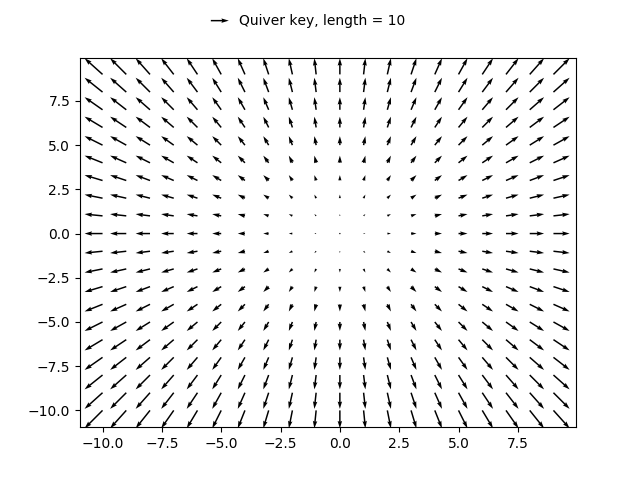You are reading an old version of the documentation (v3.0.0). For the latest version see https://matplotlib.org/stable/gallery/images_contours_and_fields/quiver_simple_demo.html

# Quiver Simple Demo¶

A simple example of a `quiver` plot with a `quiverkey`.

For more advanced options refer to Demonstration of advanced quiver and quiverkey functions.

```import matplotlib.pyplot as plt
import numpy as np

X = np.arange(-10, 10, 1)
Y = np.arange(-10, 10, 1)
U, V = np.meshgrid(X, Y)

fig, ax = plt.subplots()
q = ax.quiver(X, Y, U, V)
ax.quiverkey(q, X=0.3, Y=1.1, U=10,
label='Quiver key, length = 10', labelpos='E')

plt.show()
```